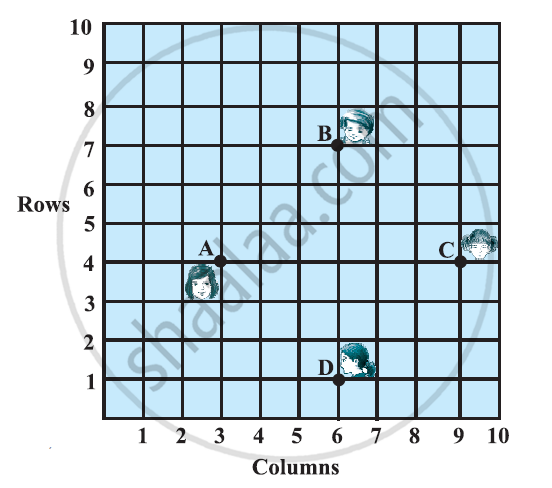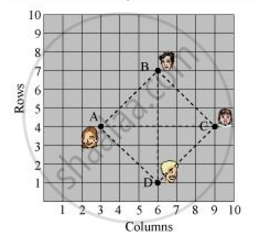# In a classroom, 4 friends are seated at the points A, B, C and D as shown in the following figure. Champa and Chameli walk into the class and after observing for a few minutes Champa asks Chameli, “Don’t you think ABCD is a square - Mathematics

In a classroom, 4 friends are seated at the points A, B, C and D as shown in the following figure. Champa and Chameli walk into the class and after observing for a few minutes Champa asks Chameli, “Don’t you think ABCD is a square?” Chameli disagrees.

Using distance formula, find which of them is correct.#### Solution

It can be observed that A (3, 4), B (6, 7), C (9, 4), and D (6, 1) are the positions of these 4 friends.

AB = sqrt((3-6)^2+(4-7)^2) = sqrt((-3)^2+(-3)^2) = sqrt(9+9) = sqrt18 = 3sqrt2

BC = sqrt((6-9)^2+(7-4)^2) = sqrt((-3)^2+(3)^2) = sqrt(9+9) = sqrt18 = 3sqrt2

CD = sqrt((9-6)^2+(4-1)^2) = sqrt((3)^2+(3)^2) = sqrt(9+9) = sqrt18 =3sqrt2

AD = sqrt((3-6)^2+(4-1)^2) = sqrt((-3)^2 + (3)^2) = sqrt(9+9) = sqrt18 = 3sqrt2

Diagonal AC=sqrt((3-9)^2+(4-4)^2)=sqrt((-6)^2)=6

Diagonal BD=sqrt((6-6)^2+(7-1)^2)=sqrt((6)^2)=6It can be observed that all sides of this quadrilateral ABCD are of the same length and also the diagonals are of the same length.

Therefore, ABCD is a square and hence, Champa was correct

Concept: Distance Formula
Is there an error in this question or solution?

#### APPEARS IN

NCERT Class 10 Maths
Chapter 7 Coordinate Geometry
Exercise 7.1 | Q 5 | Page 161